Higgs Boson

Physicist Daniela Bortoletto on the discovery of the Higgs Boson, the Large Electron-Positron collider, and the Standard Model of particle physics

faq | November 17, 2016

The Higgs boson is a particle that was hypothesized in 1964 by Peter Higgs and other theoretical physicists who were trying to understand the origin of the mass of fundamental particles. We know from elementary physics that the mass of an object is the resistance it offers to have its motion changed as described by Newton’s law F=ma. At a microscopic level, the mass of an object, like an apple, comes from its constituent molecules and atoms, which are themselves built from fundamental particles, electrons and quarks. But where do the masses of these fundamental particles come from? The theoretical work to answer this question, that appears simple, has led to the Higgs boson.

Higgs Boson and Quantum Field Theory

To describe the behavior of elementary particles, physicists use Quantum Field Theory which is a theoretical framework that is based both on relativity and quantum mechanics. Both these theories are needed to describe the elementary particle physics since elementary particles are tiny (in fact we treat them as point-like) and they travel almost at the speed of light. In the 1960s, the theoretical physicists who developed what is called the Standard Model of particle physics encountered a puzzle trying to describe what is called the weak interaction. The weak interaction is the interaction responsible for nuclear fission and the hydrogen cycle in the sun that allows life on our planet. They found that if they assumed that all particles were massless, then all equations were working well and they were able to describe in a unified way both the electromagnetic and the weak interaction.

The resulting framework was not only mathematically elegant but also explained the experimental data. Nonetheless, they had a fundamental problem. The electromagnetic interaction is exchanged by a photon which is massless and it is described by 1/r^2 behavior. Therefore, this interaction can have an impact also on large scales. The weak interaction instead is active only on the nuclear scale and therefore the particles that exchange this interaction, which we denote as the W and Z boson, should have a mass of about 100 GeV (100 times the mass of a proton). But, when theoretical physicists tried to introduce mass for the W and Z bosons in their equations they did not get the correct answer any longer.Image: Particles of the Standard Model of Physics / dullhunk (flickr.com)

In 1964 Peter Higgs and other theorists realized that the solution to this problem was, in fact, to avoid putting these mass terms directly in the equations. Instead, to maintain the symmetry present in the equation, they assumed that all of space is uniformly filled with an invisible substance, which we call the Higgs field. This field exerts a drag force on particles when they accelerate through it. This resistance to the increase of the acceleration of a particle is the particle’s mass. As an analogy, we can think about moving an object in the water. When you try to move an object in water the object feels more massive than it does outside of water. The interaction of the object with the water has the effect to increase the effective mass of the object. This is what happens to particles in the Higgs field.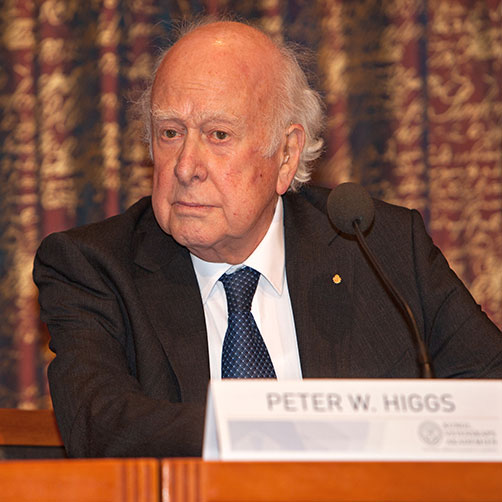Image: Peter Higgs. Nobel Laureates press conference at the Royal Swedish Academy of Sciences. December 2013 / Wikimedia Commons

In 1964, Higgs submitted a paper bringing forward this revolutionary concept to a physics journal which rejected it. Other papers were published the same year and by the 80s the Higgs mechanisms were fully accepted by the physics community. Nonetheless, to test this idea and to confirm experimentally the existence of the Higgs field permeating the whole universe, the Higgs boson had to be found. In quantum field theory, every field can be excited and this state can sometimes look like a particle, and in other cases, it can act like a wave. A particle like an electron is an excitation of the electron field. Similarly, the Higgs field can be excited by the interaction of two protons fields.

Discovery of the Higgs Boson

We found the Higgs boson in 2012 only 4 years ago. Why did it take so long to discover this particle? Finding the Higgs boson was not an easy task since the standard model of elementary particles predicts all properties of these particles but not their mass. For any given mass, the properties of the Higgs could be calculated, but the mass itself could only be determined experimentally. We only knew that the Higgs boson could not be arbitrarily heavy and that its mass should be below 2000 GeV. We also knew that since the Higgs gives mass to particles it tends to interact more with heavier particles. In fact, the most efficient way to excite the Higgs field and produce the Higgs boson would be by colliding top quarks, since top quark is the most massive known elementary particle (in fact it is as massive as an atom of gold). This of course is not possible since top quarks decay extremely fast. The Higgs boson also decays preferentially to the heaviest particles it can decay to, once conservation of energy and momentum is taken into account. Therefore, it is quite challenging to produce the Higgs boson and furthermore, if you do not know its mass you will not know how it would decay. This made the search for this particle quite complex. We were truly looking for a needle in a haystack.

Many searches for the Higgs boson took place after 1964. Some of the most important searches were performed at CERN’s Large Electron-Positron (LEP) collider. This is a collider in which electrons and positrons, circulating in opposite directions are smashed together, which operated in the same tunnel that is now used for the Large Hadron Collider (LHC). LEP reached maximum energy of 209 GeV while the LHC delivered 13,000 GeV, overs 60 times the energy of LEP, during its latest run.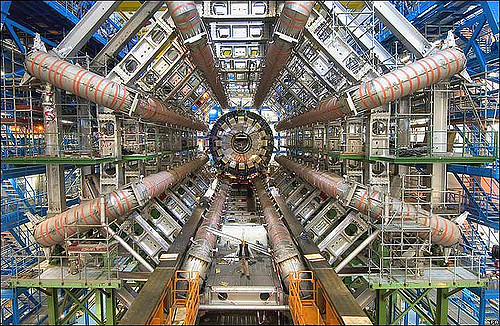Image: The Large Hadron Collider / Image Editor (flickr.com)

Electron-positron colliders present some advantages with respect to protons colliders. In electron-positron colliders, two fundamental particles, the electron and positron, are smashed together. Instead in proton-proton or a proton anti-proton colliders, we are smashing together the ensembles of quarks, antiquarks, and gluons that make up protons and antiprotons. Therefore, the collision at electron-positron collisions provides a cleaner, easier environment than proton-proton collisions for experimentalists. Furthermore, in electron-positron colliders, the exact energy of the colliding particles is known, and this provides important information for the analysis of events.

At LEP all the energy could be used to create Higgs boson particles. Nonetheless, since the mass of electrons (and positrons) is very low, their probability of interacting with the Higgs field and creating Higgs bosons is negligible. Fortunately, Higgs bosons have a large coupling to Z bosons which can be copiously produced at LEP. This allowed to search for the Higgs boson first by looking to a Z boson decay to a Higgs and a pair of pairs of leptons (electron, muons). Such decays were not observed at LEP and therefore we concluded that the mass of the Higgs boson had to be larger than 58 GeV. Sometimes at LEP you can also produce what is called a virtual boson, this is a Z boson which energy and momentum do not add up to the Z mass of 91 GeV. A virtual Z boson is an excitation of the Z quantum field that decays very rapidly and can produce a Z boson together with a Higgs boson. By 2000, when LEP was turned off, experimentalists were able to determine that the Higgs boson’s mass should be larger than 114 GeV. This was very close to the maximum mass that could be probed which was equal to the peak energy of the machine of 209 GeV minus the Z mass of 91 Gev which is yields 118 GeV.

In fact, when LEP was turned off there was a possible hint consistent with a Higgs boson of 115 GeV. Nonetheless, the machine was turned off to allow the construction and installation of the Large Hadron Collider in the same tunnel. At that point, the search for the Higgs moved to the Tevatron, which was the highest energy collider operating at the time. The Tevatron was located at Fermi National Laboratory (Fermilab). At the Tevatron protons collided with anti-protons at a peak energy of almost 2000 GeV. Both protons and antiprotons are mainly made up of gluons and the lightest quarks (up and down quarks) and antiquarks (anti-up and anti-down). Gluons are massless and therefore do not couple directly to the Higgs field. Nonetheless, sometimes the gluons field can be excited in high energy collisions to produce a top quark field. Then the top quark fields can interact with the Higgs field and create Higgs bosons. This indirect mechanism, called gluon fusion, accounts for most Higgs bosons produced in proton-proton or proton-antiproton collider. Another mechanism, called vector boson fusion, is due to the fact that high-energy protons (or antiprotons) can create pairs of W and Z bosons, which then interact to create a Higgs boson. In associated production, a Higgs boson is produced together with a Z or a W boson.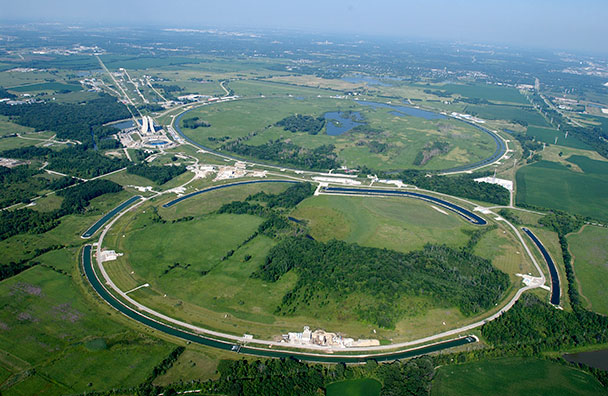Image: Fermi National Accelerator Laboratory, Main Ring and Main Injector as seen from the air / wikipedia.org

Since the Higgs has a tiny lifetime of only 10-22 seconds, to detect it we must reconstruct the particles it decays into. It is interesting to note that if the Higgs boson greater than twice the mass of the W and Z boson it will decay predominately into a pair of these gauge bosons. In fact, the Tevatron was able to exclude the existence of Higgs boson with a mass between 165 and 175 GeV since, if the Higgs had such a mass, it should have decayed into a W pair and it would have been observed at the Tevatron. If the Higgs is heavier than twice the top quark mass, then the Higgs would preferentially decay into a top quark pair. The most difficult range to find the Higgs boson is when it has a mass between 115 GeV and about 150 GeV. The highest branching fraction for a Higgs with a mass below 135 GeV is into a pair of b-quarks. Unfortunately, it is very difficult to reconstruct the decay of a Higgs boson into a pair of b-quarks since we cannot reconstruct the energy of the b-quarks very precisely and pair of b-quarks are produced in enormous quantities through other Standard Model processes. By the end of its run in 2010 the Tevatron established the evidence for Higgs decays into a pair of b-quarks at the 3 sigma level which was not high enough to claim a discovery.

The collider that finally allowed us to find the Higgs is the Large Hadron Collider (LHC). This accelerator is located in a 27-kilometers long underground circular tunnel located near Geneva and crossing the border between Switzerland and France. About 9,000 superconducting magnets steer bunches of protons in this racetrack, cycling around in opposite directions. Each bunch contains 100 billion protons travelling almost at the speed of light. At these speeds, the protons go around the tunnel about 11,000 times each second. They are steered by magnets to collide in caverns equipped by giant detectors which can decide extremely rapidly which collision is interesting and should be recorded. The LHC was built with the aim of discovering the Higgs boson. The collision of protons at the LHC started at an energy of 7 trillion electron volts (TeV) and they are now reaching record energy of 13 TeV. Two of the detectors operating at the LHC were especially designed to discover the Higgs boson, in the mass range predicted by the standard model.

The LHC had two major advantages over the Tevatron. In its first run, the LHC reached a record an energy four times higher than the Tevatron (and now 6.5 time more). At the higher energies of the LHC the production rate of the Higgs boson is much larger than at the Tevatron. The LHC also achieves higher collision rates and therefore more Higgs bosons can be produced. Therefore, the LHC was able to probe very rare decay modes of the Higgs. As we now know the Higgs boson has a mass of 125 GeV. A Higgs boson of this mass can decay to a pair of photons. Photons are massless and do not interact with the Higgs field. Top quarks carry electric charge and therefore they can interact with photons. Top quarks also interact with the Higgs field since they are very massive. Therefore, the Higgs boson can decay indirectly into two photons, through top quarks, but the probability for this decay is only 0.23 per cent.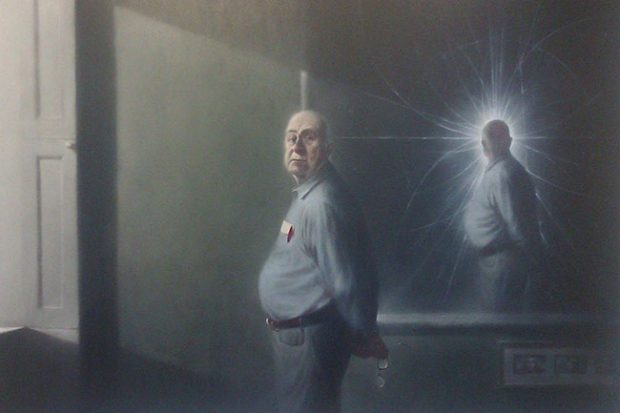Image: Peter Higgs portrait by Ken Currie, University of Edinburgh / dullhunk (flickr.com)

Nonetheless, this decay is very important since it yields a very clean signature. By measuring the energy and momentum of the two photons one can determine the existence of the Higgs boson by observing an increase of events at the mass of the Higgs above a smooth background. Higgs bosons in this mass range can also decay into a “real” W or Z and a “virtual” W and Z, but these decays are less frequent than if the Higgs was heavy enough to decay into two real particles. Just to give you an order of magnitude only 1 in 300 Higgs decays to two Z particles, and a Higgs particle lighter than about 130 GeV only decays to two Z particles about 1.5 percent of the time. Then the Z boson has a very distinctive decay into an electron and a positron or a positive and a negative muon which can easily detected in the experiment. By combining the 2 photons, the 2 Z, and the 2 W decay channels both ATLAS and CMS were able to reach the 5 sigma significance that is required in particle physics experiments to claim a discovery.

The discovery of the Higgs boson was announced on July 4, 2012. I was at CERN and I witnessed the excitement at the lab by watching the presentations given by the spokesperson of ATLAS and CMS from the council chamber at CERN with members of the press. It was a true triumph for theoretical and experimental particle physics.

ATLAS and CMS Experiments

Since then, the two experiments, ATLAS and CMS, collected 4 times more data at 8,000 GeV, during the first run of the LHC, called Run1. During 2014 to 2015, the LHC was upgraded to reach an energy of 13,000 GeV and the experiments have already collected more than the data that we had during run 1. We have already determined many properties of the Higgs boson. The rate of the Higgs decay into W and Z bosons, which are spin 1 particle, has been measured very precisely and it agrees with the predictions of the SM. We know that the Higgs couples to fermions, spin ½ particles such as quarks and leptons since we know indirectly that it couples indirectly to top quark through its production by gluon fusion and its decay to photons. We have also observed the decay of the Higgs into tau leptons but the decay into two b-quarks remains unobserved. Studies of the decay of Higgs show that the Higgs is a spin zero particle. All measurements made up to now agree with the standard model nonetheless we have probed only the coupling to quarks and leptons belonging to the third generation of quarks and leptons. There is still more work to do.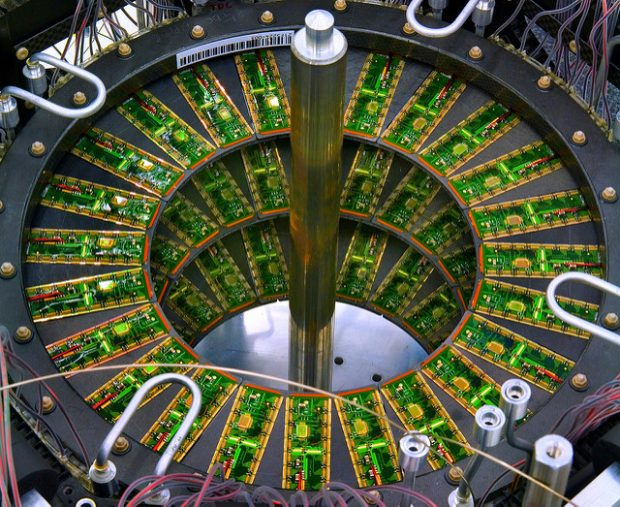Image: ATLAS Experiment Searches / berkeleylab (flickr.com)

LHC and Future Directions of Research

Up to now, we have collected only about 2% of the data that we expect to collect at the LHC and its planned upgrade called the HL-LHC. In the standard model, we have 3 generations of quarks and leptons. By now we have probed only the coupling to quarks and leptons in the third generation, which is more massive. More data is needed to probe the couplings to quark and leptons in the second generation of quarks and leptons. We also hope that measurements of the Higgs can bring surprises. For example, the Higgs might couple to invisible particles and provide a window to the discovery of the dark matter particles.Physicist Mahdi Godazgar on the arrow of time, Schrödinger equation, and Einstein's relativity theory
With more data we should also be able to measure the interaction of the Higgs boson with itself and therefore determine the shape of the Higgs potential. This is extremely interesting since it could reveal if our universe has evolved to a minimum of the Higgs potential or if there are other minima. This measurement could tell us if the Universe is stable, or if is “metastable”, which means that it is stable on cosmological time scales but ultimately headed towards a collapse. The current measured mass of the top quark and the Higgs bosons, seem to indicate, that if there is no new physics up to the Planck scale, the universe is in a metastable state. This means that the minimum of the Higgs potential is a local minimum and not a global minimum. Nonetheless, the most recent analysis concluded that it is possible for the Universe to be fully stable if the true values of measured parameters are only 1.3 standard deviations away from the current best estimates. More precise measurements of the Higgs boson mass, the top-quark mass, the strong coupling constant, and other parameters will help to answer this question.

Open Questions in Particle Physics

Finding the Higgs boson has completed the standard model of elementary particles but many questions remain unanswered. Furthermore, the Higgs boson itself has open new questions. The most striking question is why the Higgs mass has a mass of only 125 GeV. The Higgs boson is a spin 0 particle which interacts through virtual loops with most particles and gains mass through these interactions. Therefore, it very difficult for the Higgs boson to remain light without invoking an additional symmetry. This is called the hierarchy problem. Supersymmetry offers an elegant solution to this problem by postulating the existence of a new symmetry between bosons, which have an integer spin and fermions which have a half-integer spin. According to this symmetry for each spin 1/2 fermion in the standard model, like the top quark, there is a supersymmetric particle with integer spin, the stop. Similarly, for each boson in the SM there is a supersymmetric particle with spin ½. The contributions of SM particles to the Higgs mass is cancelled by the contributions of its super-partner. Supersymmetry is also interesting because it provides a particle, the lightest supersymmetric particle, which could explain the dark matter observed in the universe.Professor of Physics, ATLAS Oxford Group, University of Oxford
Did you like it? Share it with your friends!
Published items
0779
To be published soon
+92

Most viewed

New